Dynamical Systems Commons™

279 Full-Text Articles 362 Authors 62,170 Downloads53 Institutions

All Articles in Dynamical Systems

279 full-text articles. Page 4 of 12.

Predator-Prey Dynamics With Intraspecific Competition And An Allee Effect In The Predator Population, 2016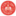The New School

Predator-Prey Dynamics With Intraspecific Competition And An Allee Effect In The Predator Population, Anne E. Yust, Erin N. Bodine

Annual Symposium on Biomathematics and Ecology: Education and Research

No abstract provided.

2016University of Dayton

On The Perfect Reconstruction Of The Structure Of Dynamic Networks, Alan Veliz-Cuba

Annual Symposium on Biomathematics and Ecology: Education and Research

No abstract provided.

2016Unity College

Comparing The Effects Of General And Selective Culling On Chronic Wasting Disease (Cwd) Prevalence, Elliott J. Moran

Annual Symposium on Biomathematics and Ecology: Education and Research

No abstract provided.

2016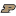Southeast University

Dem-Cfd Numerical Simulation And Experimental Validation Of Heat Transfer And Two-Component Flow In Fluidized Bed, Feihong Guo

The 8th International Conference on Physical and Numerical Simulation of Materials Processing

No abstract provided.

2016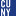The Graduate Center, City University of New York

The Fourth Movement Of György Ligeti's Piano Concerto: Investigating The Musical-Mathematical Connection, Cynthia L. Wong

All Dissertations, Theses, and Capstone Projects

This interdisciplinary study explores musical-mathematical analogies in the fourth movement of Ligeti’s Piano Concerto. Its aim is to connect musical analysis with the piece’s mathematical inspiration. For this purpose, the dissertation is divided into two sections. Part I (Chapters 1-2) provides musical and mathematical context, including an explanation of ideas related to Ligeti’s mathematical inspiration. Part II (Chapters 3-5) delves into an analysis of the rhythm, form, melody / motive, and harmony. Appendix A is a reduced score of the entire movement, labeled according to my analysis.

Computing The Optimal Path In Stochastic Dynamical Systems, 2016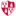Montclair State University

Computing The Optimal Path In Stochastic Dynamical Systems, Martha Bauver, Eric Forgoston, Lora Billings

Department of Mathematical Sciences Faculty Scholarship and Creative Works

In stochastic systems, one is often interested in finding the optimal path that maximizes the probability of escape from a metastable state or of switching between metastable states. Even for simple systems, it may be impossible to find an analytic form of the optimal path, and in high- dimensional systems, this is almost always the case. In this article, we formulate a constructive methodology that is used to compute the optimal path numerically. The method utilizes finite-time Lyapunov exponents, statistical selection criteria, and a Newton-based iterative minimizing scheme. The method is applied to four examples. The first example is a ...

Computing The Optimal Path In Stochastic Dynamical Systems, 2016Montclair State University

Computing The Optimal Path In Stochastic Dynamical Systems, Martha Bauver, Eric Forgoston, Lora Billings

Lora Billings

In stochastic systems, one is often interested in finding the optimal path that maximizes the probability of escape from a metastable state or of switching between metastable states. Even for simple systems, it may be impossible to find an analytic form of the optimal path, and in high- dimensional systems, this is almost always the case. In this article, we formulate a constructive methodology that is used to compute the optimal path numerically. The method utilizes finite-time Lyapunov exponents, statistical selection criteria, and a Newton-based iterative minimizing scheme. The method is applied to four examples. The first example is a ...

Computing The Optimal Path In Stochastic Dynamical Systems, 2016Montclair State University

Computing The Optimal Path In Stochastic Dynamical Systems, Martha Bauver, Eric Forgoston, Lora Billings

Eric Forgoston

In stochastic systems, one is often interested in finding the optimal path that maximizes the probability of escape from a metastable state or of switching between metastable states. Even for simple systems, it may be impossible to find an analytic form of the optimal path, and in high- dimensional systems, this is almost always the case. In this article, we formulate a constructive methodology that is used to compute the optimal path numerically. The method utilizes finite-time Lyapunov exponents, statistical selection criteria, and a Newton-based iterative minimizing scheme. The method is applied to four examples. The first example is a ...

Anthrax Models Involving Immunology, Epidemiology And Controls, 2016University of Tennessee, Knoxville

Anthrax Models Involving Immunology, Epidemiology And Controls, Buddhi Raj Pantha

Doctoral Dissertations

This dissertation is divided in two parts. Chapters 2 and 3 consider the use of optimal control theory in an anthrax epidemiological model. Models consisting system of ordinary differential equations (ODEs) and partial differential differential equations (PDEs) are considered to describe the dynamics of infection spread. Two controls, vaccination and disposal of infected carcasses, are considered and their optimal management strategies are investigated. Chapter 4 consists modeling early host pathogen interaction in an inhalational anthrax infection which consists a system of ODEs that describes early dynamics of bacteria-phagocytic cell interaction associated to an inhalational anthrax infection.

First we consider a ...

Open And Dense Topological Transitivity Of Extensions By Non-Compact Fiber Of Hyperbolic Systems: A Review, 2016West Chester University of Pennsylvania

Open And Dense Topological Transitivity Of Extensions By Non-Compact Fiber Of Hyperbolic Systems: A Review, Viorel Nitica, Andrei Török

Viorel Nitica

Currently, there is great renewed interest in proving the topological transitivity of various classes of continuous dynamical systems. Even though this is one of the most basic dynamical properties that can be investigated, the tools used by various authors are quite diverse and are strongly related to the class of dynamical systems under consideration. The goal of this review article is to present the state of the art for the class of Hölder extensions of hyperbolic systems with non-compact connected Lie group fiber. The hyperbolic systems we consider are mostly discrete time. In particular, we address the stability and genericity ...

Open And Dense Topological Transitivity Of Extensions By Non-Compact Fiber Of Hyperbolic Systems: A Review, 2016West Chester University of Pennsylvania

Open And Dense Topological Transitivity Of Extensions By Non-Compact Fiber Of Hyperbolic Systems: A Review, Viorel Nitica, Andrei Török

Viorel Nitica

Currently, there is great renewed interest in proving the topological transitivity of various classes of continuous dynamical systems. Even though this is one of the most basic dynamical properties that can be investigated, the tools used by various authors are quite diverse and are strongly related to the class of dynamical systems under consideration. The goal of this review article is to present the state of the art for the class of Hölder extensions of hyperbolic systems with non-compact connected Lie group fiber. The hyperbolic systems we consider are mostly discrete time. In particular, we address the stability and genericity ...

Signal Velocity In Oscillator Arrays, 2016Portland State University

Signal Velocity In Oscillator Arrays, Carlos E. Cantos, David K. Hammond, J. J. P. Veerman

J. J. P. Veerman

We investigate a system of coupled oscillators on the circle, which arises from a simple model for behavior of large numbers of autonomous vehicles. The model considers asymmetric, linear, decentralized dynamics, where the acceleration of each vehicle depends on the relative positions and velocities between itself and a set of local neighbors. We first derive necessary and sufficient conditions for asymptotic stability, then derive expressions for the phase velocity of propagation of disturbances in velocity through this system. We show that the high frequencies exhibit damping, which implies existence of well-defined signal velocities c+>0 and c−f(x−c ...

On Rank Driven Dynamical Systems, 2016Portland State University

On Rank Driven Dynamical Systems, J. J. P. Veerman, F. J. Prieto

J. J. P. Veerman

We investigate a class of models related to the Bak-Sneppen model, initially proposed to study evolution. The BS model is extremely simple and yet captures some forms of “complex behavior” such as self-organized criticality that is often observed in physical and biological systems. In this model, random fitnesses in [0, 1] are associated to agents located at the vertices of a graph G. Their fitnesses are ranked from worst (0) to best (1). At every time-step the agent with the worst fitness and some others with a priori given rank probabilities are replaced by new agents with random fitnesses. We ...

An Expansion Property Of Boolean Linear Maps, 2016Shanghai Jiao Tong University

An Expansion Property Of Boolean Linear Maps, Yaokun Wu, Zeying Xu, Yinfeng Zhu

Electronic Journal of Linear Algebra

Given a finite set $K$, a Boolean linear map on $K$ is a map $f$ from the set $2^K$ of all subsets of $K$ into itself with $f(\emptyset )=\emptyset$ such that $f(A\cup B)=f(A)\cup f(B)$ holds for all $A,B\in 2^K$. For fixed subsets $X, Y$ of $K$, to predict if $Y$ is reachable from $X$ in the dynamical system driven by $f$, one can assume the existence of nonnegative integers $h$ with $f^h(X)=Y$, find an upper bound $\alpha$ for the minimum of all such assumed integers \$h ...

A Bi-Stable Switch In Virus Dynamics Can Explain The Differences In Disease Outcome Following Siv Infections In Rhesus Macaques, 2016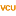Virginia Tech

A Bi-Stable Switch In Virus Dynamics Can Explain The Differences In Disease Outcome Following Siv Infections In Rhesus Macaques, Stanca Ciupe, Christopher Miller, Jonathan Forde

Biology and Medicine Through Mathematics Conference

No abstract provided.

Growth Dynamics For Pomacea Maculata, 2016University of Louisiana at Lafayette

Growth Dynamics For Pomacea Maculata, Lihong Zhao, Karyn L. Sutton, Jacoby Carter

Biology and Medicine Through Mathematics Conference

No abstract provided.

2016State University of New York at New Paltz

Wilson-Cowan Coupled Dynamics In A Model Of The Cortico-Striato-Thalamo-Cortical Circuit, Anca R. Radulescu

Biology and Medicine Through Mathematics Conference

No abstract provided.

Robust Traveling Waves In Chains Of Simple Neural Oscillators, 2016The Cooper Union for the Advancement of Science and Art

Robust Traveling Waves In Chains Of Simple Neural Oscillators, Stanislav M. Mintchev

Biology and Medicine Through Mathematics Conference

No abstract provided.

Clustering-Based Robot Navigation And Control, 2016University of Pennsylvania

Clustering-Based Robot Navigation And Control, Omur Arslan, Dan P. Guralnik, Daniel E. Koditschek

Departmental Papers (ESE)

In robotics, it is essential to model and understand the topologies of configuration spaces in order to design provably correct motion planners. The common practice in motion planning for modelling configuration spaces requires either a global, explicit representation of a configuration space in terms of standard geometric and topological models, or an asymptotically dense collection of sample configurations connected by simple paths. In this short note, we present an overview of our recent results that utilize clustering for closing the gap between these two complementary approaches. Traditionally an unsupervised learning method, clustering offers automated tools to discover hidden intrinsic structures ...

2016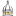The University of Southern Mississippi

Applications Of The Sierpiński Triangle To Musical Composition, Samuel C. Dent

Honors Theses

The present paper builds on the idea of composing music via fractals, specifically the Sierpiński Triangle and the Sierpiński Pedal Triangle. The resulting methods are intended to produce not just a series of random notes, but a series that we think pleases the ear. One method utilizes the iterative process of generating the Sierpiński Triangle and Sierpiński Pedal Triangle via matrix operations by applying this process to a geometric configuration of note names. This technique designs the largest components of the musical work first, then creates subsequent layers where each layer adds more detail.